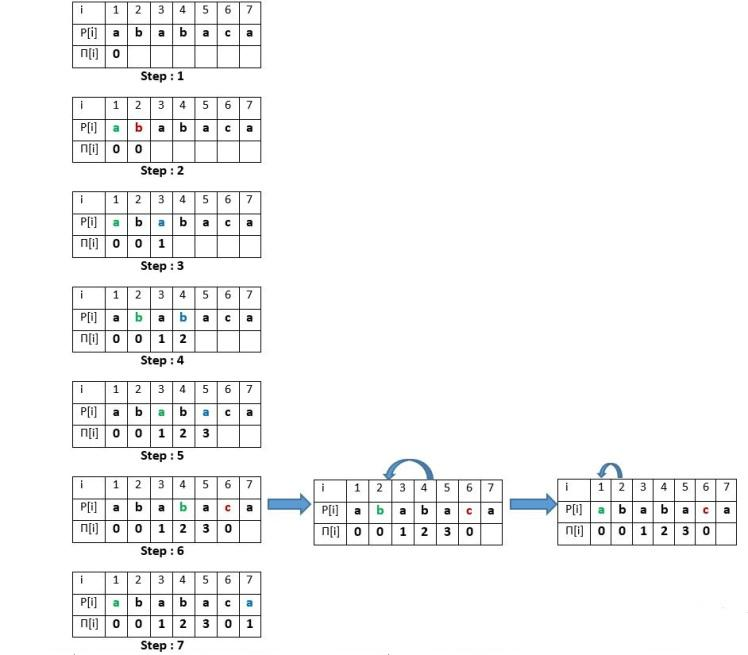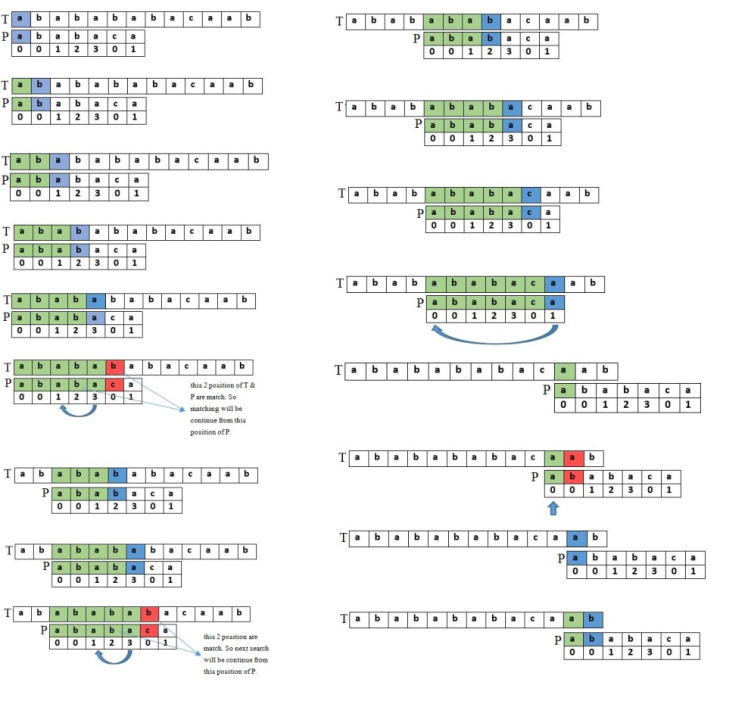# Knuth–Morris–Pratt(KMP) Algorithm

String is an inevitable part in programming. In large sphere, it is essential element in computer science. Searching for a specific string or matching a string is one of the common task in computer science related work. I will discuss about one of the linear time string searching algorithm - Knuth–Morris–Pratt algorithm, in short KMP algorithm.

### Background

Lets think of a scenario - We have a String `S = "we will rock you"` and we need to find another string `A = "rock"` in S. What is the first approach to do this task? The most easiest one would be- starting from the 0-index of string S to find the find the first character of A. If it matches then check the next characters of A in S. The solution in ruby will be like -

``````def first_string_search s, a
i = 0
s_length = s.size
a_length = a.size

while i < s_length do
next if s[i] != a
j = 1
while i+j < s_length && j < a_length && s[i+j] == a[j] do
j+= 1
return i if j == a_length
end
i+= 1
end
false
end
``````

if you observe the code, u can see that the complexity of this solution is O(mn) where m = length of s and n = length of a. Its Ok if both s and a is small in size. but what if they are very big. Obviously the system will hang. Just think we can find any string in PDF reader or notepad or sublime in no time, no matter how big the opened content is(may be 1000 page book). If they would use this approoach it would take a day. Now we will learn about KMP algorithm which solves this time issue and thus do efficient search.

### Knuth–Morris–Pratt Algorithm

in 1977, Donald Knuth, Vaughan Pratt and James H. Morris published an algorithm for string search which is known as KMP algorithm. In previous solution, we tried to match each letter of a string. but in KMP algorithm it is tried to skip some letter- it is the basic idea

Algorithm steps are as follows

• At first we need to make a Pi table in terms of patter string P.
• After that we will check for patterns by the help of Pi table
• If any character is not matched, we can skip some characters based on pi table

### Pi Table

This table is the helping array for KMP algorithm. The size of this table will be the size of pattern string P. Each element of this array will store the matched longest prefix or suffix of that string P.If you look at the picture above - for the first index of P the suffix and prefix is empty so we put 0 in that index.

• For second index the scenario is same.
• For third element of P, suffix = prefix = 1. So we put 1
• For fourth element of P, suffix = prefix = "ab" = 2. So we put 2 and so on

If we do it on code

``````def pi_table pattern
f=0
j = 0
m = pattern.size
for i = 1; i < m; i++ do
if pattern[i]==pattern[j]
f[i]=j+1
j += 1
else
while j!=0 do
j = f[j-1]
if pattern[i]==pattern[j]
f[i] = j+1
j+= 1
break
end
end
end
end
end
``````

### Find Pattern using Pi table

Now we need to to find the pattern string P in reference string S. You can see the picture belowFinding a pattern in string S using the pi table is just like making the pi table above. If we find a mismatch in S and P, we can skip some character in S using pi table because we already matched the suffix and prefix. If we code this in ruby, we can write like this -

``````def kmp text, pattern
j = 0
for i = 0; i < n; i++ do
if text[i]==pattern[j]
if j==m-1
return true if j==m-1
j+= 1
else
while j!=0 do
j = f[j-1]
if text[i]==pattern[j]
j+= 1
break
end
end
end
end
false
end
``````

### Complexity

For the pi table computation, the for loop is iterated m-1 times where m = size of pattern string P. The complexity of that part is `O(m)`. And in KMP matching part, the loop runs n times where n = size of text S. So the time complexity for matching part is `O(n)`. and the time complexity for whole KMP algorithm is `O(m+n)`, which is far better than naive O(m*n). The space complexity of this algorithm is `O(m)`.